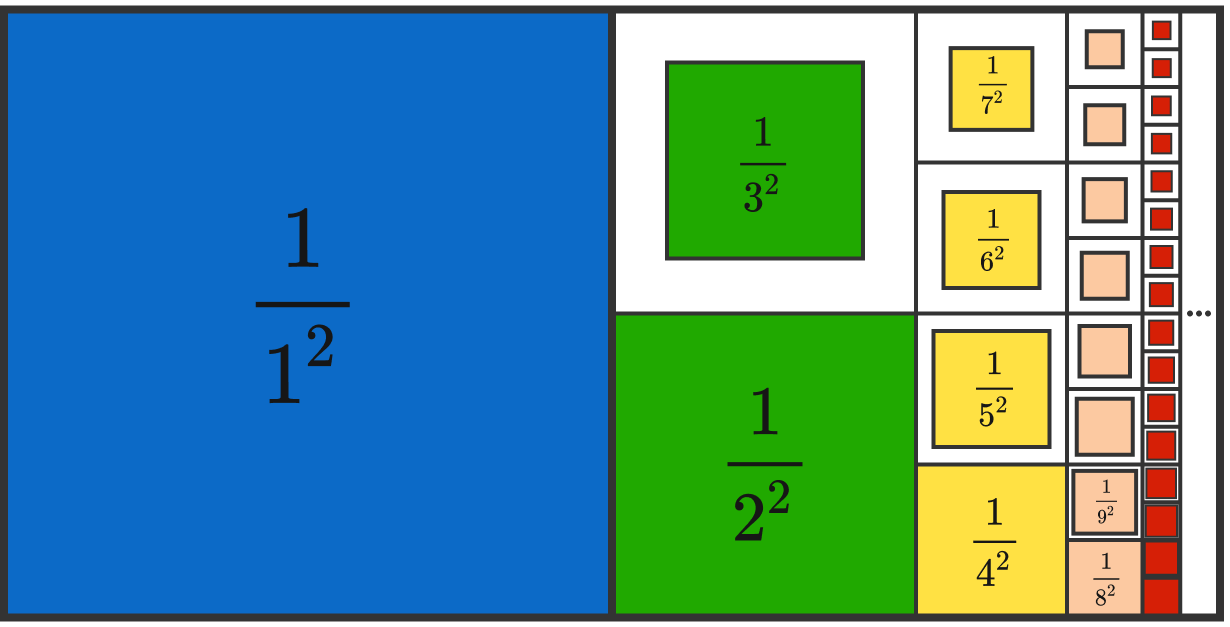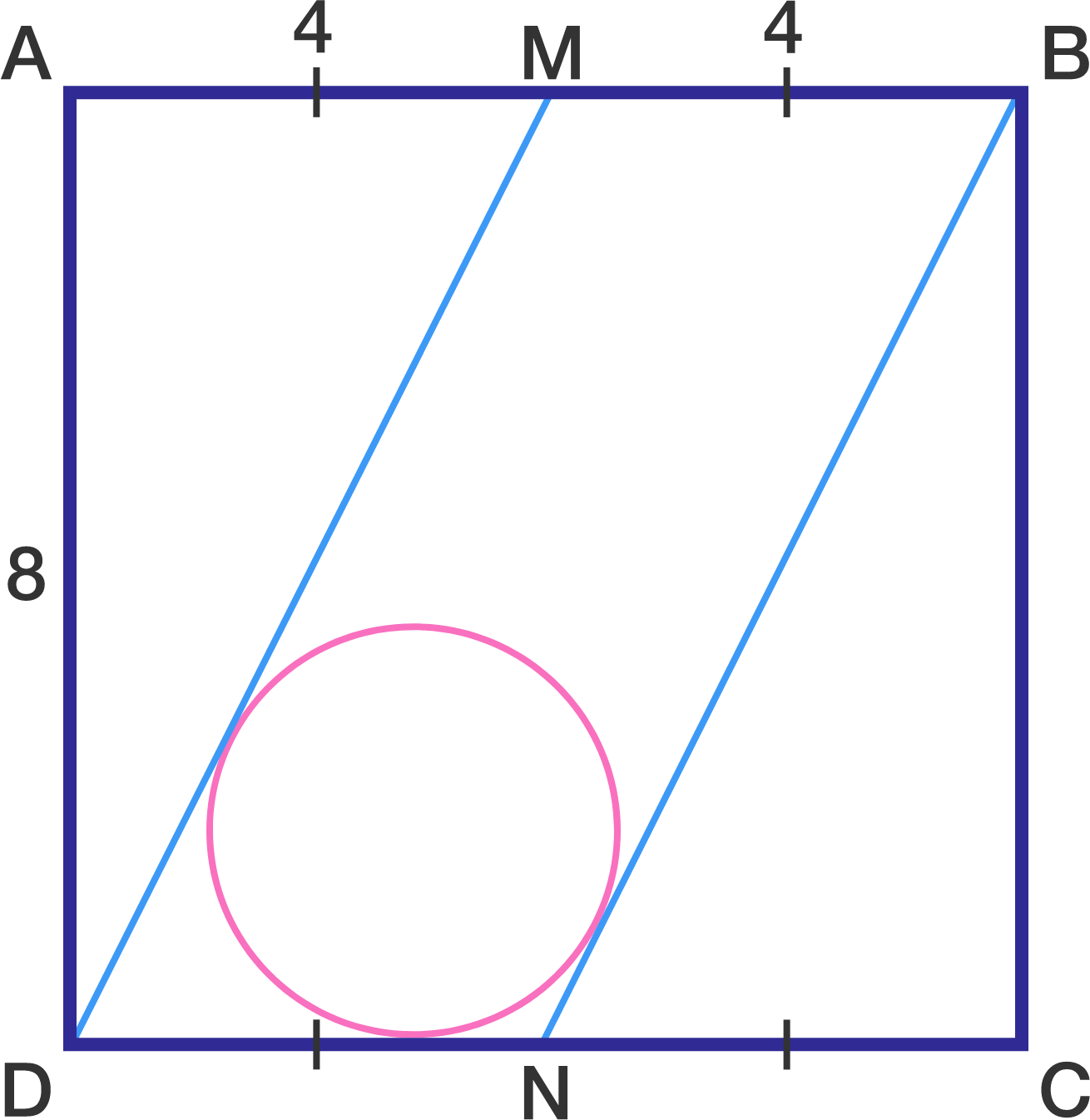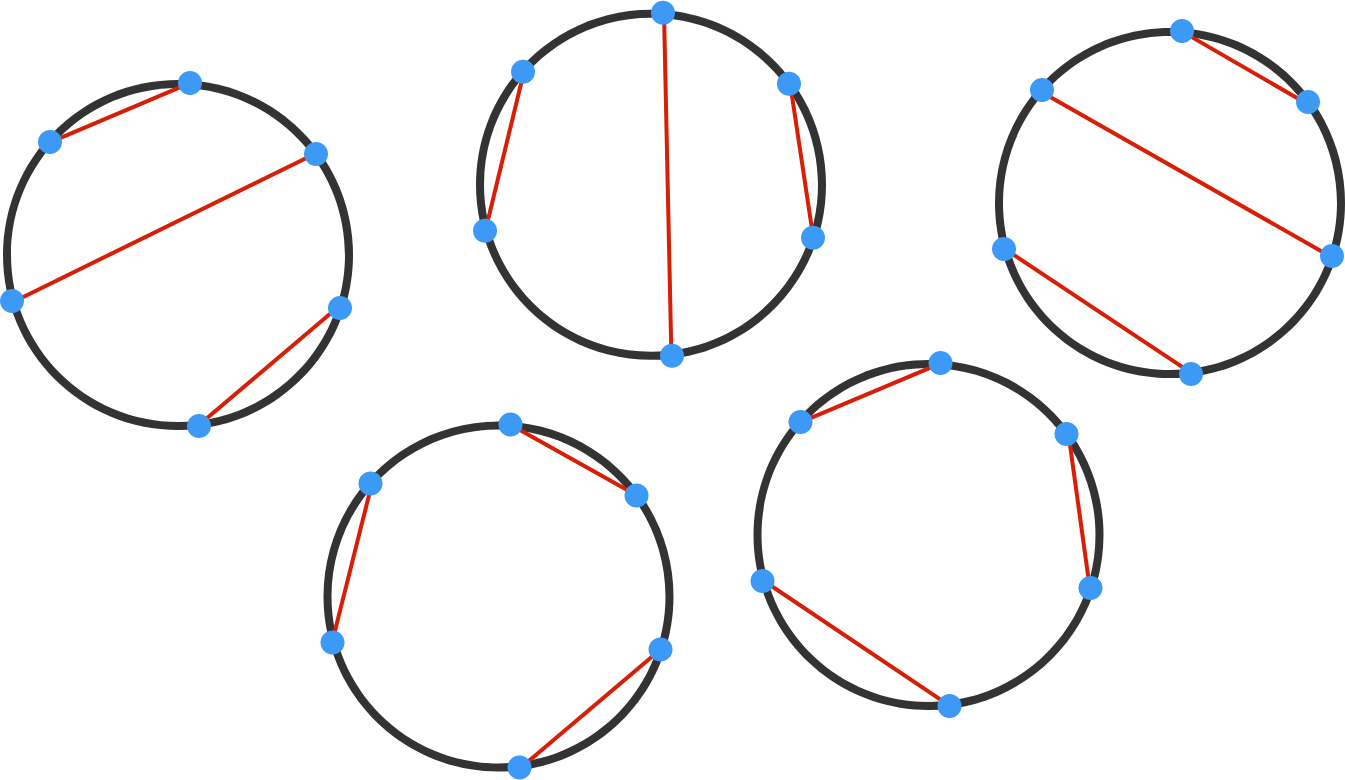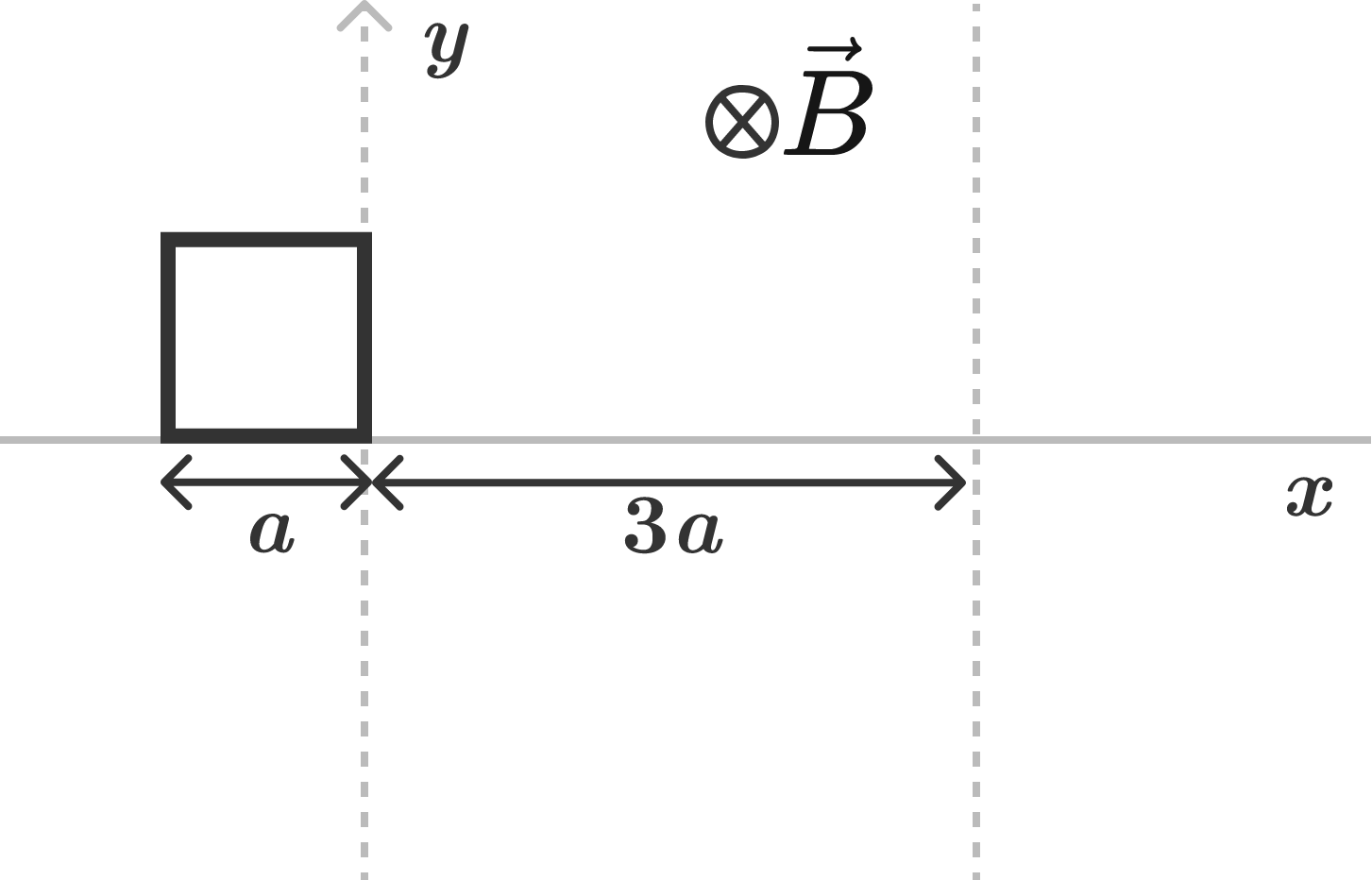# Problems of the Week

Contribute a problem

# 2017-02-13 IntermediateWhat mathematical fact is demonstrated above?

$\begin{array} { c c c c c } \square & + & \square & = & \square \\ \square & + & \square & = & \square \\ \square & + & \square & = & \square \\ \square & + & \square & = & \square \\ \square & + & \square & = & \square \\ \square & + & \square & = & \square \\ \end{array}$ Is it possible to fill the above squares with all the integers from 1 to 18 (using each number only once), such that each equation holds true?

For example, if we had only 4 rows and 12 numbers from 1 to 12 available, we could do the following:
$\begin{array} { c c c c c } 1 &+& 6 & =& 7 \\ 2 &+& 10 & =& 12 \\ 3 &+& 8 & =& 11 \\ 4 &+& 5 & =& 9. \end{array}$The above shows a square ABCD with side length 8.
M and N are the midpoints of sides AB and CD, respectively.
A circle is inscribed in between lines DM and NB and the bottom side of the square.

Find the radius of this circle to 3 decimal places.

We label 10 points on the circumference of a circle as $P_1, P_2, \ldots, P_{10}.$ Find the number of ways to connect the points in pairs with non-intersecting chords, with no points left unconnected.


Hint: When the number of points is 6, the number of ways is 5, as shown below.A square wire loop having side length $a$, mass $m$, and resistance $R$ is moving along the positive $x$-axis at a speed of $v_0$. It enters a uniform, steady magnetic field $\vec B = B_0 \big(-\widehat k \big)$ at $t = 0$ seconds, as shown in the figure.

Find the total amount of heat loss in the resistance.

Details and Assumptions:

• The magnitude of $v_0$ is sufficient that the loop comes out of the region of magnetic field with some speed.

• Neglect any type of energy loss other than the heat loss in resistance of the wires of the square.

• Take $v_0 = \dfrac{3B^2 a^3}{mR}$.

×

Problem Loading...

Note Loading...

Set Loading...